Anúncio

# Python_Module_1.pdf

Assist Proff at ALva's Institute of Engineering and Technology em R.K.College of engg & Tech
25 de Mar de 2023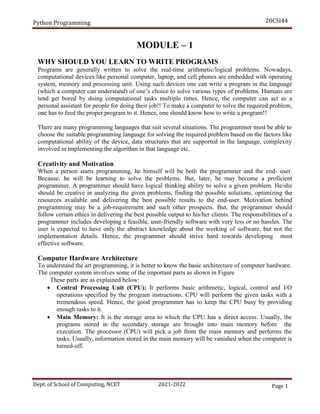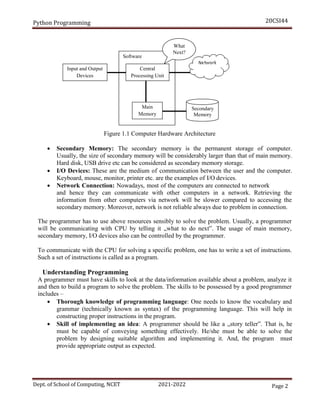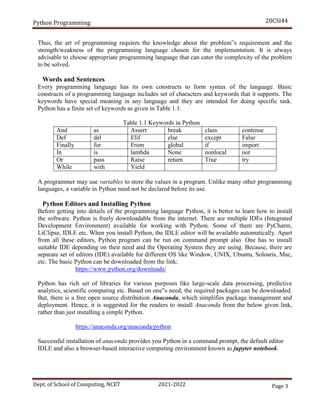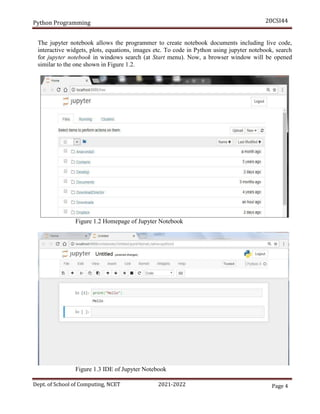Anúncio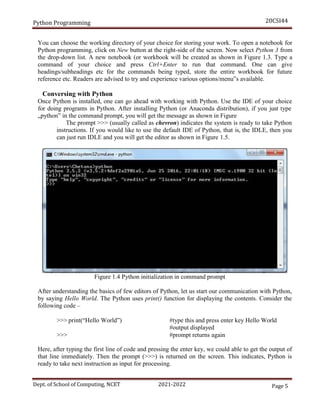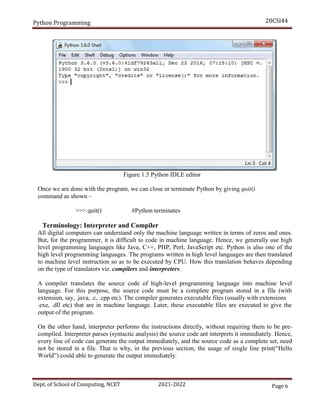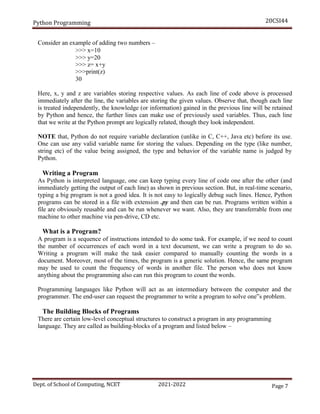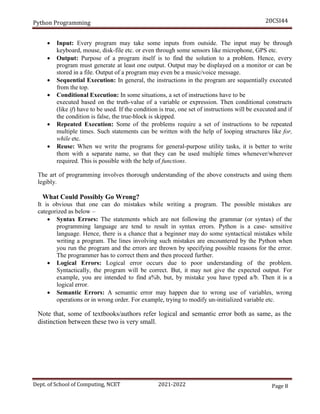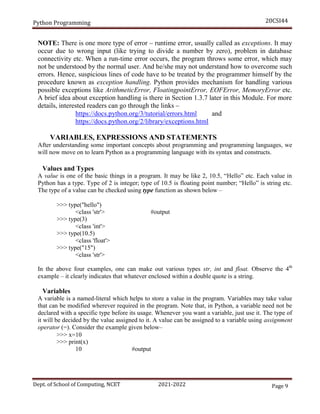Anúncio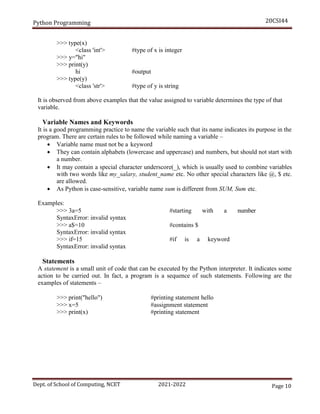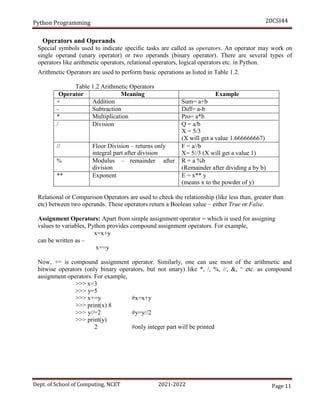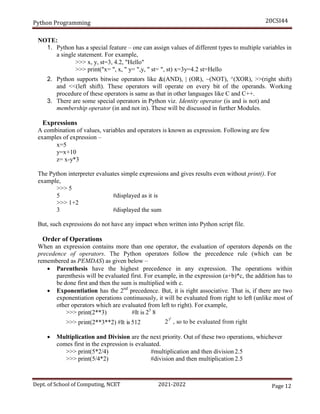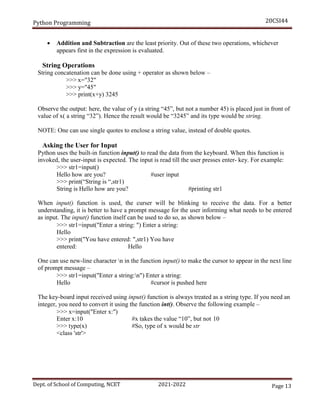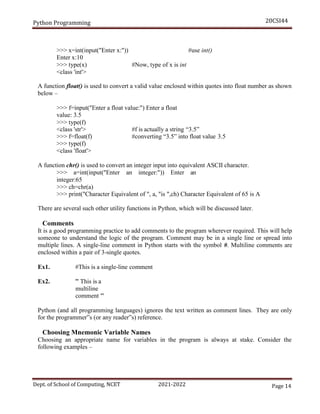Anúncio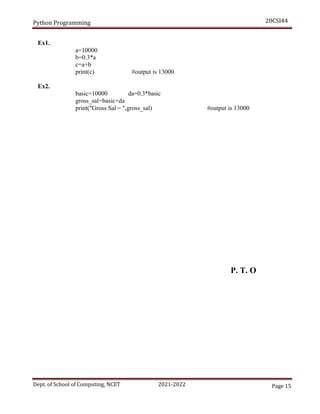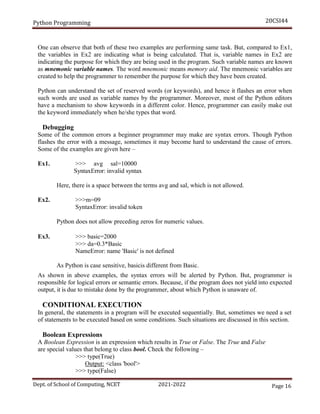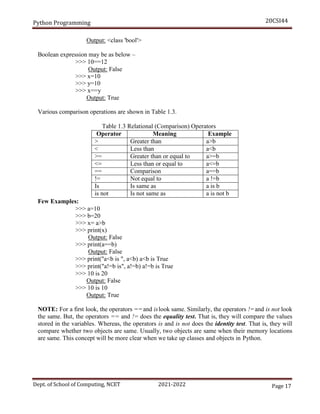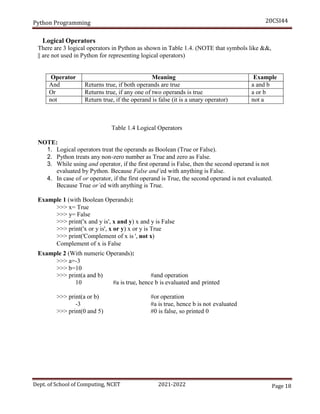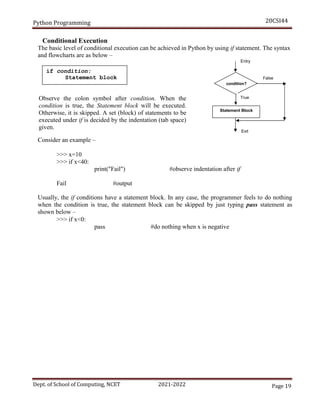Anúncio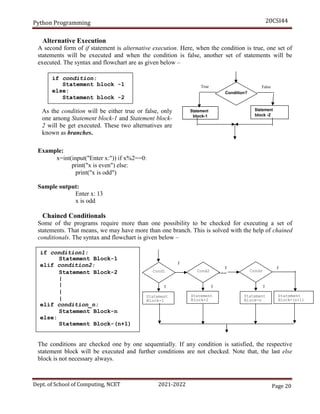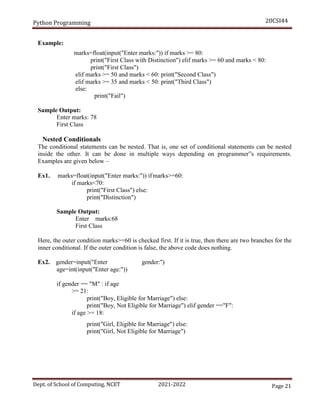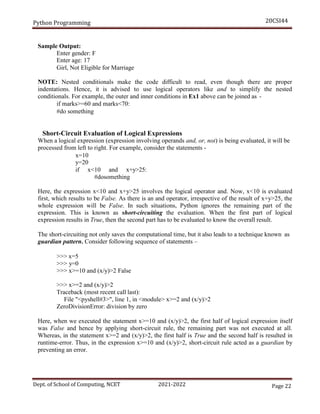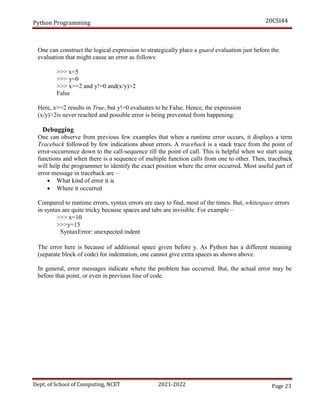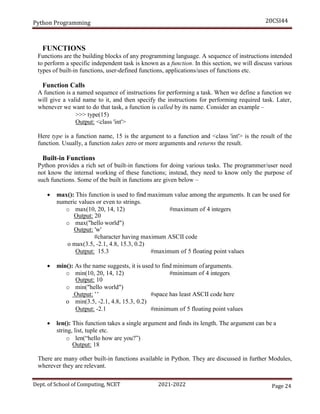Anúncio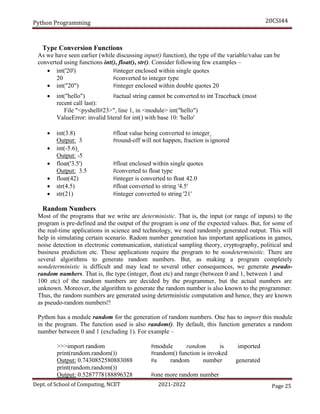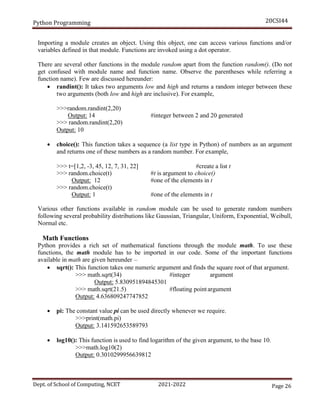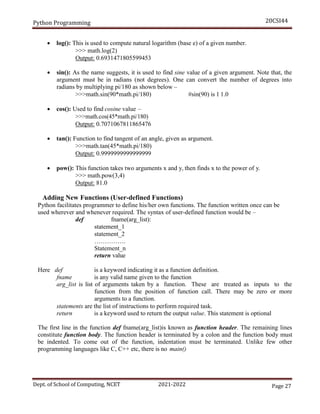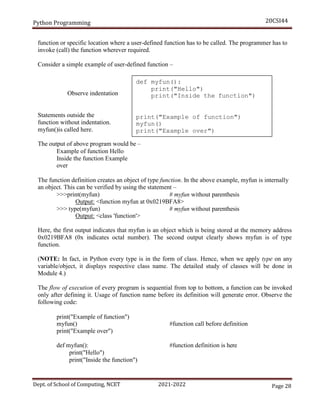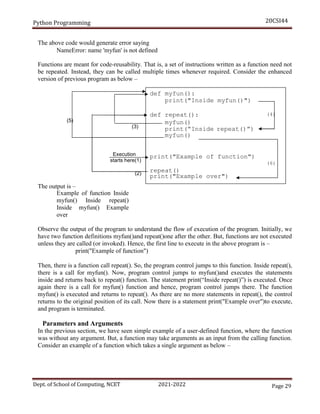Anúncio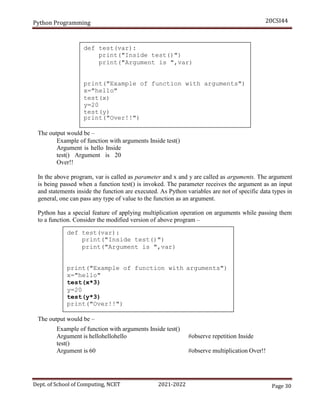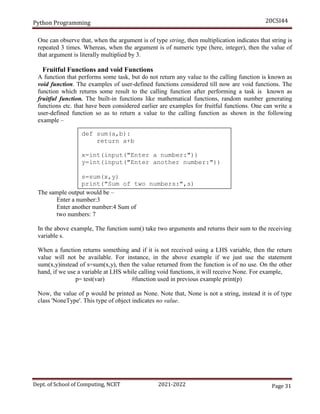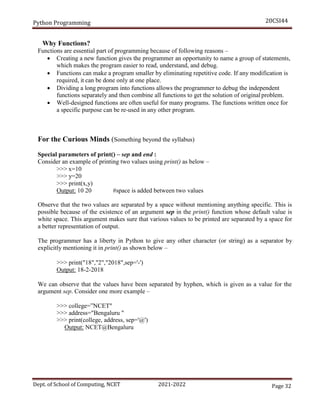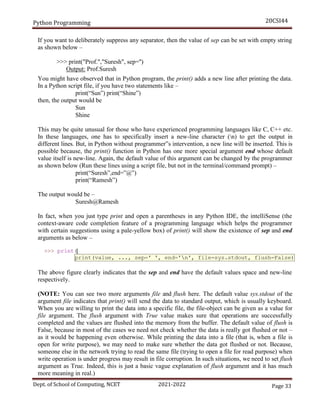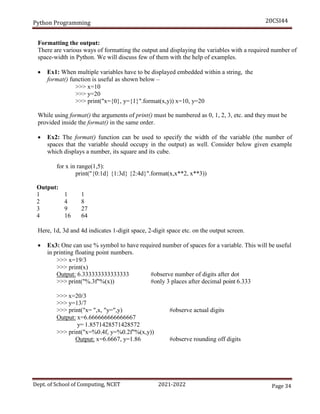Próximos SlideShares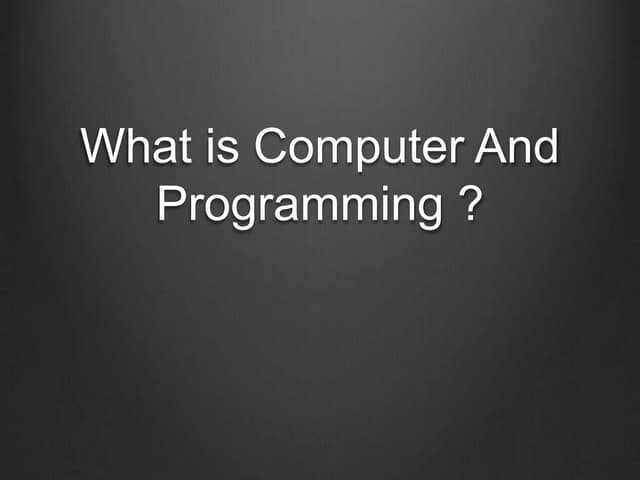Computer and Programming
Carregando em ... 3
1 de 34
Anúncio

### Python_Module_1.pdf

1. Python Programming 20CSI44 Dept. of School of Computing, NCET 2021-2022 Page 1 MODULE – 1 WHY SHOULD YOU LEARN TO WRITE PROGRAMS Programs are generally written to solve the real-time arithmetic/logical problems. Nowadays, computational devices like personal computer, laptop, and cell phones are embedded with operating system, memory and processing unit. Using such devices one can write a program in the language (which a computer can understand) of one’s choice to solve various types of problems. Humans are tend get bored by doing computational tasks multiple times. Hence, the computer can act as a personal assistant for people for doing their job!! To make a computer to solve the required problem, one has to feed the proper program to it. Hence, one should know how to write a program!! There are many programming languages that suit several situations. The programmer must be able to choose the suitable programming language for solving the required problem based on the factors like computational ability of the device, data structures that are supported in the language, complexity involved in implementing the algorithm in that language etc. Creativity and Motivation When a person starts programming, he himself will be both the programmer and the end- user. Because, he will be learning to solve the problems. But, later, he may become a proficient programmer. A programmer should have logical thinking ability to solve a given problem. He/she should be creative in analyzing the given problems, finding the possible solutions, optimizing the resources available and delivering the best possible results to the end-user. Motivation behind programming may be a job-requirement and such other prospects. But, the programmer should follow certain ethics in delivering the best possible output to his/her clients. The responsibilities of a programmer includes developing a feasible, user-friendly software with very less or no hassles. The user is expected to have only the abstract knowledge about the working of software, but not the implementation details. Hence, the programmer should strive hard towards developing most effective software. Computer Hardware Architecture To understand the art programming, it is better to know the basic architecture of computer hardware. The computer system involves some of the important parts as shown in Figure These parts are as explained below:  Central Processing Unit (CPU): It performs basic arithmetic, logical, control and I/O operations specified by the program instructions. CPU will perform the given tasks with a tremendous speed. Hence, the good programmer has to keep the CPU busy by providing enough tasks to it.  Main Memory: It is the storage area to which the CPU has a direct access. Usually, the programs stored in the secondary storage are brought into main memory before the execution. The processor (CPU) will pick a job from the main memory and performs the tasks. Usually, information stored in the main memory will be vanished when the computer is turned-off.
2. Python Programming 20CSI44 Dept. of School of Computing, NCET 2021-2022 Page 2 Figure 1.1 Computer Hardware Architecture  Secondary Memory: The secondary memory is the permanent storage of computer. Usually, the size of secondary memory will be considerably larger than that of main memory. Hard disk, USB drive etc can be considered as secondary memory storage.  I/O Devices: These are the medium of communication between the user and the computer. Keyboard, mouse, monitor, printer etc. are the examples of I/O devices.  Network Connection: Nowadays, most of the computers are connected to network and hence they can communicate with other computers in a network. Retrieving the information from other computers via network will be slower compared to accessing the secondary memory. Moreover, network is not reliable always due to problem in connection. The programmer has to use above resources sensibly to solve the problem. Usually, a programmer will be communicating with CPU by telling it „what to do next‟. The usage of main memory, secondary memory, I/O devices also can be controlled by the programmer. To communicate with the CPU for solving a specific problem, one has to write a set of instructions. Such a set of instructions is called as a program. Understanding Programming A programmer must have skills to look at the data/information available about a problem, analyze it and then to build a program to solve the problem. The skills to be possessed by a good programmer includes –  Thorough knowledge of programming language: One needs to know the vocabulary and grammar (technically known as syntax) of the programming language. This will help in constructing proper instructions in the program.  Skill of implementing an idea: A programmer should be like a „story teller‟. That is, he must be capable of conveying something effectively. He/she must be able to solve the problem by designing suitable algorithm and implementing it. And, the program must provide appropriate output as expected. Software What Next? Secondary Memory Main Memory Central Processing Unit Input and Output Devices
4. Python Programming 20CSI44 Dept. of School of Computing, NCET 2021-2022 Page 4 The jupyter notebook allows the programmer to create notebook documents including live code, interactive widgets, plots, equations, images etc. To code in Python using jupyter notebook, search for jupyter notebook in windows search (at Start menu). Now, a browser window will be opened similar to the one shown in Figure 1.2. Figure 1.2 Homepage of Jupyter Notebook Figure 1.3 IDE of Jupyter Notebook
6. Python Programming 20CSI44 Dept. of School of Computing, NCET 2021-2022 Page 6 Figure 1.5 Python IDLE editor Once we are done with the program, we can close or terminate Python by giving quit() command as shown – >>> quit() #Python terminates Terminology: Interpreter and Compiler All digital computers can understand only the machine language written in terms of zeros and ones. But, for the programmer, it is difficult to code in machine language. Hence, we generally use high level programming languages like Java, C++, PHP, Perl, JavaScript etc. Python is also one of the high level programming languages. The programs written in high level languages are then translated to machine level instruction so as to be executed by CPU. How this translation behaves depending on the type of translators viz. compilers and interpreters. A compiler translates the source code of high-level programming language into machine level language. For this purpose, the source code must be a complete program stored in a file (with extension, say, .java, .c, .cpp etc). The compiler generates executable files (usually with extensions .exe, .dll etc) that are in machine language. Later, these executable files are executed to give the output of the program. On the other hand, interpreter performs the instructions directly, without requiring them to be pre- compiled. Interpreter parses (syntactic analysis) the source code ant interprets it immediately. Hence, every line of code can generate the output immediately, and the source code as a complete set, need not be stored in a file. That is why, in the previous section, the usage of single line print(“Hello World”) could able to generate the output immediately.
7. Python Programming 20CSI44 Dept. of School of Computing, NCET 2021-2022 Page 7 Consider an example of adding two numbers – >>> x=10 >>> y=20 >>> z= x+y >>>print(z) 30 Here, x, y and z are variables storing respective values. As each line of code above is processed immediately after the line, the variables are storing the given values. Observe that, though each line is treated independently, the knowledge (or information) gained in the previous line will be retained by Python and hence, the further lines can make use of previously used variables. Thus, each line that we write at the Python prompt are logically related, though they look independent. NOTE that, Python do not require variable declaration (unlike in C, C++, Java etc) before its use. One can use any valid variable name for storing the values. Depending on the type (like number, string etc) of the value being assigned, the type and behavior of the variable name is judged by Python. Writing a Program As Python is interpreted language, one can keep typing every line of code one after the other (and immediately getting the output of each line) as shown in previous section. But, in real-time scenario, typing a big program is not a good idea. It is not easy to logically debug such lines. Hence, Python programs can be stored in a file with extension .py and then can be run. Programs written within a file are obviously reusable and can be run whenever we want. Also, they are transferrable from one machine to other machine via pen-drive, CD etc. What is a Program? A program is a sequence of instructions intended to do some task. For example, if we need to count the number of occurrences of each word in a text document, we can write a program to do so. Writing a program will make the task easier compared to manually counting the words in a document. Moreover, most of the times, the program is a generic solution. Hence, the same program may be used to count the frequency of words in another file. The person who does not know anything about the programming also can run this program to count the words. Programming languages like Python will act as an intermediary between the computer and the programmer. The end-user can request the programmer to write a program to solve one‟s problem. The Building Blocks of Programs There are certain low-level conceptual structures to construct a program in any programming language. They are called as building-blocks of a program and listed below –
8. Python Programming 20CSI44 Dept. of School of Computing, NCET 2021-2022 Page 8  Input: Every program may take some inputs from outside. The input may be through keyboard, mouse, disk-file etc. or even through some sensors like microphone, GPS etc.  Output: Purpose of a program itself is to find the solution to a problem. Hence, every program must generate at least one output. Output may be displayed on a monitor or can be stored in a file. Output of a program may even be a music/voice message.  Sequential Execution: In general, the instructions in the program are sequentially executed from the top.  Conditional Execution: In some situations, a set of instructions have to be executed based on the truth-value of a variable or expression. Then conditional constructs (like if) have to be used. If the condition is true, one set of instructions will be executed and if the condition is false, the true-block is skipped.  Repeated Execution: Some of the problems require a set of instructions to be repeated multiple times. Such statements can be written with the help of looping structures like for, while etc.  Reuse: When we write the programs for general-purpose utility tasks, it is better to write them with a separate name, so that they can be used multiple times whenever/wherever required. This is possible with the help of functions. The art of programming involves thorough understanding of the above constructs and using them legibly. What Could Possibly Go Wrong? It is obvious that one can do mistakes while writing a program. The possible mistakes are categorized as below –  Syntax Errors: The statements which are not following the grammar (or syntax) of the programming language are tend to result in syntax errors. Python is a case- sensitive language. Hence, there is a chance that a beginner may do some syntactical mistakes while writing a program. The lines involving such mistakes are encountered by the Python when you run the program and the errors are thrown by specifying possible reasons for the error. The programmer has to correct them and then proceed further.  Logical Errors: Logical error occurs due to poor understanding of the problem. Syntactically, the program will be correct. But, it may not give the expected output. For example, you are intended to find a%b, but, by mistake you have typed a/b. Then it is a logical error.  Semantic Errors: A semantic error may happen due to wrong use of variables, wrong operations or in wrong order. For example, trying to modify un-initialized variable etc. Note that, some of textbooks/authors refer logical and semantic error both as same, as the distinction between these two is very small.
9. Python Programming 20CSI44 Dept. of School of Computing, NCET 2021-2022 Page 9 NOTE: There is one more type of error – runtime error, usually called as exceptions. It may occur due to wrong input (like trying to divide a number by zero), problem in database connectivity etc. When a run-time error occurs, the program throws some error, which may not be understood by the normal user. And he/she may not understand how to overcome such errors. Hence, suspicious lines of code have to be treated by the programmer himself by the procedure known as exception handling. Python provides mechanism for handling various possible exceptions like ArithmeticError, FloatingpointError, EOFError, MemoryError etc. A brief idea about exception handling is there in Section 1.3.7 later in this Module. For more details, interested readers can go through the links – https://docs.python.org/3/tutorial/errors.html and https://docs.python.org/2/library/exceptions.html VARIABLES, EXPRESSIONS AND STATEMENTS After understanding some important concepts about programming and programming languages, we will now move on to learn Python as a programming language with its syntax and constructs. Values and Types A value is one of the basic things in a program. It may be like 2, 10.5, “Hello” etc. Each value in Python has a type. Type of 2 is integer; type of 10.5 is floating point number; “Hello” is string etc. The type of a value can be checked using type function as shown below – >>> type("hello") <class 'str'> #output >>> type(3) <class 'int'> >>> type(10.5) <class 'float'> >>> type("15") <class 'str'> In the above four examples, one can make out various types str, int and float. Observe the 4th example – it clearly indicates that whatever enclosed within a double quote is a string. Variables A variable is a named-literal which helps to store a value in the program. Variables may take value that can be modified wherever required in the program. Note that, in Python, a variable need not be declared with a specific type before its usage. Whenever you want a variable, just use it. The type of it will be decided by the value assigned to it. A value can be assigned to a variable using assignment operator (=). Consider the example given below– >>> x=10 >>> print(x) 10 #output
10. Python Programming 20CSI44 Dept. of School of Computing, NCET 2021-2022 Page 10 >>> type(x) <class 'int'> #type of x is integer >>> y="hi" >>> print(y) hi #output >>> type(y) <class 'str'> #type of y is string It is observed from above examples that the value assigned to variable determines the type of that variable. Variable Names and Keywords It is a good programming practice to name the variable such that its name indicates its purpose in the program. There are certain rules to be followed while naming a variable –  Variable name must not be a keyword  They can contain alphabets (lowercase and uppercase) and numbers, but should not start with a number.  It may contain a special character underscore(_), which is usually used to combine variables with two words like my_salary, student_name etc. No other special characters like @, \$ etc. are allowed.  As Python is case-sensitive, variable name sum is different from SUM, Sum etc. Examples: >>> 3a=5 #starting with a number SyntaxError: invalid syntax >>> a\$=10 #contains \$ SyntaxError: invalid syntax >>> if=15 #if is a keyword SyntaxError: invalid syntax Statements A statement is a small unit of code that can be executed by the Python interpreter. It indicates some action to be carried out. In fact, a program is a sequence of such statements. Following are the examples of statements – >>> print("hello") #printing statement hello >>> x=5 #assignment statement >>> print(x) #printing statement
11. Python Programming 20CSI44 Dept. of School of Computing, NCET 2021-2022 Page 11 Operators and Operands Special symbols used to indicate specific tasks are called as operators. An operator may work on single operand (unary operator) or two operands (binary operator). There are several types of operators like arithmetic operators, relational operators, logical operators etc. in Python. Arithmetic Operators are used to perform basic operations as listed in Table 1.2. Table 1.2 Arithmetic Operators Operator Meaning Example + Addition Sum= a+b - Subtraction Diff= a-b * Multiplication Pro= a*b / Division Q = a/b X = 5/3 (X will get a value 1.666666667) // Floor Division – returns only integral part after division F = a//b X= 5//3 (X will get a value 1) % Modulus division – remainder after R = a %b (Remainder after dividing a by b) ** Exponent E = x** y (means x to the powder of y) Relational or Comparison Operators are used to check the relationship (like less than, greater than etc) between two operands. These operators return a Boolean value – either True or False. Assignment Operators: Apart from simple assignment operator = which is used for assigning values to variables, Python provides compound assignment operators. For example, x=x+y can be written as – x+=y Now, += is compound assignment operator. Similarly, one can use most of the arithmetic and bitwise operators (only binary operators, but not unary) like *, /, %, //, &, ^ etc. as compound assignment operators. For example, >>> x=3 >>> y=5 >>> x+=y #x=x+y >>> print(x) 8 >>> y//=2 #y=y//2 >>> print(y) 2 #only integer part will be printed
12. Python Programming 20CSI44 Dept. of School of Computing, NCET 2021-2022 Page 12 NOTE: 1. Python has a special feature – one can assign values of different types to multiple variables in a single statement. For example, >>> x, y, st=3, 4.2, "Hello" >>> print("x= ", x, " y= ",y, " st= ", st) x=3y=4.2 st=Hello 2. Python supports bitwise operators like &(AND), | (OR), ~(NOT), ^(XOR), >>(right shift) and <<(left shift). These operators will operate on every bit of the operands. Working procedure of these operators is same as that in other languages like C and C++. 3. There are some special operators in Python viz. Identity operator (is and is not) and membership operator (in and not in). These will be discussed in further Modules. Expressions A combination of values, variables and operators is known as expression. Following are few examples of expression – x=5 y=x+10 z= x-y*3 The Python interpreter evaluates simple expressions and gives results even without print(). For example, >>> 5 5 #displayed as it is >>> 1+2 3 #displayed the sum But, such expressions do not have any impact when written into Python script file. Order of Operations When an expression contains more than one operator, the evaluation of operators depends on the precedence of operators. The Python operators follow the precedence rule (which can be remembered as PEMDAS) as given below –  Parenthesis have the highest precedence in any expression. The operations within parenthesis will be evaluated first. For example, in the expression (a+b)*c, the addition has to be done first and then the sum is multiplied with c.  Exponentiation has the 2nd precedence. But, it is right associative. That is, if there are two exponentiation operations continuously, it will be evaluated from right to left (unlike most of other operators which are evaluated from left to right). For example, >>> print(2**3) #It is 23 8 >>> print(2**3**2) #It is 512 232 , so to be evaluated from right  Multiplication and Division are the next priority. Out of these two operations, whichever comes first in the expression is evaluated. >>> print(5*2/4) #multiplication and then division 2.5 >>> print(5/4*2) #division and then multiplication 2.5
13. Python Programming 20CSI44 Dept. of School of Computing, NCET 2021-2022 Page 13  Addition and Subtraction are the least priority. Out of these two operations, whichever appears first in the expression is evaluated. String Operations String concatenation can be done using + operator as shown below – >>> x="32" >>> y="45" >>> print(x+y) 3245 Observe the output: here, the value of y (a string “45”, but not a number 45) is placed just in front of value of x( a string “32”). Hence the result would be “3245” and its type would be string. NOTE: One can use single quotes to enclose a string value, instead of double quotes. Asking the User for Input Python uses the built-in function input() to read the data from the keyboard. When this function is invoked, the user-input is expected. The input is read till the user presses enter- key. For example: >>> str1=input() Hello how are you? #user input >>> print(“String is “,str1) String is Hello how are you? #printing str1 When input() function is used, the curser will be blinking to receive the data. For a better understanding, it is better to have a prompt message for the user informing what needs to be entered as input. The input() function itself can be used to do so, as shown below – >>> str1=input("Enter a string: ") Enter a string: Hello >>> print("You have entered: ",str1) You have entered: Hello One can use new-line character n in the function input() to make the cursor to appear in the next line of prompt message – >>> str1=input("Enter a string:n") Enter a string: Hello #cursor is pushed here The key-board input received using input() function is always treated as a string type. If you need an integer, you need to convert it using the function int(). Observe the following example – >>> x=input("Enter x:") Enter x:10 #x takes the value “10”, but not 10 >>> type(x) #So, type of x would be str <class 'str'>
14. Python Programming 20CSI44 Dept. of School of Computing, NCET 2021-2022 Page 14 >>> x=int(input("Enter x:")) #use int() Enter x:10 >>> type(x) #Now, type of x is int <class 'int'> A function float() is used to convert a valid value enclosed within quotes into float number as shown below – >>> f=input("Enter a float value:") Enter a float value: 3.5 >>> type(f) <class 'str'> #f is actually a string “3.5” >>> f=float(f) #converting “3.5” into float value 3.5 >>> type(f) <class 'float'> A function chr() is used to convert an integer input into equivalent ASCII character. >>> a=int(input("Enter an integer:")) Enter an integer:65 >>> ch=chr(a) >>> print("Character Equivalent of ", a, "is ",ch) Character Equivalent of 65 is A There are several such other utility functions in Python, which will be discussed later. Comments It is a good programming practice to add comments to the program wherever required. This will help someone to understand the logic of the program. Comment may be in a single line or spread into multiple lines. A single-line comment in Python starts with the symbol #. Multiline comments are enclosed within a pair of 3-single quotes. Ex1. #This is a single-line comment Ex2. ''' This is a multiline comment ''' Python (and all programming languages) ignores the text written as comment lines. They are only for the programmer‟s (or any reader‟s) reference. Choosing Mnemonic Variable Names Choosing an appropriate name for variables in the program is always at stake. Consider the following examples –
15. Python Programming 20CSI44 Dept. of School of Computing, NCET 2021-2022 Page 15 Ex1. a=10000 b=0.3*a c=a+b print(c) #output is 13000 Ex2. basic=10000 da=0.3*basic gross_sal=basic+da print("Gross Sal = ",gross_sal) #output is 13000 P. T. O
16. Python Programming 20CSI44 Dept. of School of Computing, NCET 2021-2022 Page 16 One can observe that both of these two examples are performing same task. But, compared to Ex1, the variables in Ex2 are indicating what is being calculated. That is, variable names in Ex2 are indicating the purpose for which they are being used in the program. Such variable names are known as mnemonic variable names. The word mnemonic means memory aid. The mnemonic variables are created to help the programmer to remember the purpose for which they have been created. Python can understand the set of reserved words (or keywords), and hence it flashes an error when such words are used as variable names by the programmer. Moreover, most of the Python editors have a mechanism to show keywords in a different color. Hence, programmer can easily make out the keyword immediately when he/she types that word. Debugging Some of the common errors a beginner programmer may make are syntax errors. Though Python flashes the error with a message, sometimes it may become hard to understand the cause of errors. Some of the examples are given here – Ex1. >>> avg sal=10000 SyntaxError: invalid syntax Here, there is a space between the terms avg and sal, which is not allowed. Ex2. >>>m=09 SyntaxError: invalid token Python does not allow preceding zeros for numeric values. Ex3. >>> basic=2000 >>> da=0.3*Basic NameError: name 'Basic' is not defined As Python is case sensitive, basicis different from Basic. As shown in above examples, the syntax errors will be alerted by Python. But, programmer is responsible for logical errors or semantic errors. Because, if the program does not yield into expected output, it is due to mistake done by the programmer, about which Python is unaware of. CONDITIONAL EXECUTION In general, the statements in a program will be executed sequentially. But, sometimes we need a set of statements to be executed based on some conditions. Such situations are discussed in this section. Boolean Expressions A Boolean Expression is an expression which results in True or False. The True and False are special values that belong to class bool. Check the following – >>> type(True) Output: <class 'bool'> >>> type(False)
17. Python Programming 20CSI44 Dept. of School of Computing, NCET 2021-2022 Page 17 Output: <class 'bool'> Boolean expression may be as below – >>> 10==12 Output: False >>> x=10 >>> y=10 >>> x==y Output: True Various comparison operations are shown in Table 1.3. Table 1.3 Relational (Comparison) Operators Operator Meaning Example > Greater than a>b < Less than a<b >= Greater than or equal to a>=b <= Less than or equal to a<=b == Comparison a==b != Not equal to a !=b Is Is same as a is b is not Is not same as a is not b Few Examples: >>> a=10 >>> b=20 >>> x= a>b >>> print(x) Output: False >>> print(a==b) Output: False >>> print("a<b is ", a<b) a<b is True >>> print("a!=b is", a!=b) a!=b is True >>> 10 is 20 Output: False >>> 10 is 10 Output: True NOTE: For a first look, the operators ==and islook same. Similarly, the operators !=and is not look the same. But, the operators == and != does the equality test. That is, they will compare the values stored in the variables. Whereas, the operators is and is not does the identity test. That is, they will compare whether two objects are same. Usually, two objects are same when their memory locations are same. This concept will be more clear when we take up classes and objects in Python.
18. Python Programming 20CSI44 Dept. of School of Computing, NCET 2021-2022 Page 18 Logical Operators There are 3 logical operators in Python as shown in Table 1.4. (NOTE that symbols like &&, || are not used in Python for representing logical operators) Table 1.4 Logical Operators NOTE: 1. Logical operators treat the operands as Boolean (True or False). 2. Python treats any non-zero number as True and zero as False. 3. While using and operator, if the first operand is False, then the second operand is not evaluated by Python. Because False and’ed with anything is False. 4. In case of or operator, if the first operand is True, the second operand is not evaluated. Because True or’ed with anything is True. Example 1 (with Boolean Operands): >>> x= True >>> y= False >>> print('x and y is', x and y) x and y is False >>> print('x or y is', x or y) x or y is True >>> print('Complement of x is ', not x) Complement of x is False Example 2 (With numeric Operands): >>> a=-3 >>> b=10 >>> print(a and b) #and operation 10 #a is true, hence b is evaluated and printed >>> print(a or b) #or operation -3 #a is true, hence b is not evaluated >>> print(0 and 5) #0 is false, so printed 0 Operator Meaning Example And Returns true, if both operands are true a and b Or Returns true, if any one of two operands is true a or b not Return true, if the operand is false (it is a unary operator) not a
19. Python Programming 20CSI44 Dept. of School of Computing, NCET 2021-2022 Page 19 if condition: Statement block Conditional Execution The basic level of conditional execution can be achieved in Python by using if statement. The syntax and flowcharts are as below – Observe the colon symbol after condition. When the condition is true, the Statement block will be executed. Otherwise, it is skipped. A set (block) of statements to be executed under if is decided by the indentation (tab space) given. Consider an example – >>> x=10 >>> if x<40: print("Fail") #observe indentation after if Fail #output Usually, the if conditions have a statement block. In any case, the programmer feels to do nothing when the condition is true, the statement block can be skipped by just typing pass statement as shown below – >>> if x<0: pass #do nothing when x is negative Entry condition? Exit Statement Block True False
20. Python Programming 20CSI44 Dept. of School of Computing, NCET 2021-2022 Page 20 if condition1: Statement Block-1 elif condition2: Statement Block-2 | | | | elif condition_n: Statement Block-n else: Statement Block-(n+1) Statement Block-(n+1) Statement Block-n Statement Block-2 Statement Block-1 Alternative Execution A second form of if statement is alternative execution. Here, when the condition is true, one set of statements will be executed and when the condition is false, another set of statements will be executed. The syntax and flowchart are as given below – As the condition will be either true or false, only one among Statement block-1 and Statement block- 2 will be get executed. These two alternatives are known as branches. Example: x=int(input("Enter x:")) if x%2==0: print("x is even") else: print("x is odd") Sample output: Enter x: 13 x is odd Chained Conditionals Some of the programs require more than one possibility to be checked for executing a set of statements. That means, we may have more than one branch. This is solved with the help of chained conditionals. The syntax and flowchart is given below – The conditions are checked one by one sequentially. If any condition is satisfied, the respective statement block will be executed and further conditions are not checked. Note that, the last else block is not necessary always. if condition: Statement block -1 else: Statement block -2 True False Condition? Statement block-1 Statement block -2 F Cond1 Cond2 F Condn F T T T
21. Python Programming 20CSI44 Dept. of School of Computing, NCET 2021-2022 Page 21 Example: marks=float(input("Enter marks:")) if marks >= 80: print("First Class with Distinction") elif marks >= 60 and marks < 80: print("First Class") elif marks >= 50 and marks < 60: print("Second Class") elif marks >= 35 and marks < 50: print("Third Class") else: print("Fail") Sample Output: Enter marks: 78 First Class Nested Conditionals The conditional statements can be nested. That is, one set of conditional statements can be nested inside the other. It can be done in multiple ways depending on programmer‟s requirements. Examples are given below – Ex1. marks=float(input("Enter marks:")) ifmarks>=60: if marks<70: print("First Class") else: print("Distinction") Sample Output: Enter marks:68 First Class Here, the outer condition marks>=60 is checked first. If it is true, then there are two branches for the inner conditional. If the outer condition is false, the above code does nothing. Ex2. gender=input("Enter gender:") age=int(input("Enter age:")) if gender == "M" : if age >= 21: print("Boy, Eligible for Marriage") else: print("Boy, Not Eligible for Marriage") elif gender =="F": if age >= 18: print("Girl, Eligible for Marriage") else: print("Girl, Not Eligible for Marriage")
22. Python Programming 20CSI44 Dept. of School of Computing, NCET 2021-2022 Page 22 Sample Output: Enter gender: F Enter age: 17 Girl, Not Eligible for Marriage NOTE: Nested conditionals make the code difficult to read, even though there are proper indentations. Hence, it is advised to use logical operators like and to simplify the nested conditionals. For example, the outer and inner conditions in Ex1 above can be joined as - if marks>=60 and marks<70: #do something Short-Circuit Evaluation of Logical Expressions When a logical expression (expression involving operands and, or, not) is being evaluated, it will be processed from left to right. For example, consider the statements - x=10 y=20 if x<10 and x+y>25: #dosomething Here, the expression x<10 and x+y>25 involves the logical operator and. Now, x<10 is evaluated first, which results to be False. As there is an and operator, irrespective of the result of x+y>25, the whole expression will be False. In such situations, Python ignores the remaining part of the expression. This is known as short-circuiting the evaluation. When the first part of logical expression results in True, then the second part has to be evaluated to know the overall result. The short-circuiting not only saves the computational time, but it also leads to a technique known as guardian pattern. Consider following sequence of statements – >>> x=5 >>> y=0 >>> x>=10 and (x/y)>2 False >>> x>=2 and (x/y)>2 Traceback (most recent call last): File "<pyshell#3>", line 1, in <module> x>=2 and (x/y)>2 ZeroDivisionError: division by zero Here, when we executed the statement x>=10 and (x/y)>2, the first half of logical expression itself was False and hence by applying short-circuit rule, the remaining part was not executed at all. Whereas, in the statement x>=2 and (x/y)>2, the first half is True and the second half is resulted in runtime-error. Thus, in the expression x>=10 and (x/y)>2, short-circuit rule acted as a guardian by preventing an error.
23. Python Programming 20CSI44 Dept. of School of Computing, NCET 2021-2022 Page 23 One can construct the logical expression to strategically place a guard evaluation just before the evaluation that might cause an error as follows: >>> x=5 >>> y=0 >>> x>=2 and y!=0 and(x/y)>2 False Here, x>=2 results in True, but y!=0 evaluates to be False. Hence, the expression (x/y)>2is never reached and possible error is being prevented from happening. Debugging One can observe from previous few examples that when a runtime error occurs, it displays a term Traceback followed by few indications about errors. A traceback is a stack trace from the point of error-occurrence down to the call-sequence till the point of call. This is helpful when we start using functions and when there is a sequence of multiple function calls from one to other. Then, traceback will help the programmer to identify the exact position where the error occurred. Most useful part of error message in traceback are –  What kind of error it is  Where it occurred Compared to runtime errors, syntax errors are easy to find, most of the times. But, whitespace errors in syntax are quite tricky because spaces and tabs are invisible. For example – >>> x=10 >>>y=15 SyntaxError: unexpected indent The error here is because of additional space given before y. As Python has a different meaning (separate block of code) for indentation, one cannot give extra spaces as shown above. In general, error messages indicate where the problem has occurred. But, the actual error may be before that point, or even in previous line of code.
24. Python Programming 20CSI44 Dept. of School of Computing, NCET 2021-2022 Page 24 FUNCTIONS Functions are the building blocks of any programming language. A sequence of instructions intended to perform a specific independent task is known as a function. In this section, we will discuss various types of built-in functions, user-defined functions, applications/uses of functions etc. Function Calls A function is a named sequence of instructions for performing a task. When we define a function we will give a valid name to it, and then specify the instructions for performing required task. Later, whenever we want to do that task, a function is called by its name. Consider an example – >>> type(15) Output: <class 'int'> Here type is a function name, 15 is the argument to a function and <class 'int'> is the result of the function. Usually, a function takes zero or more arguments and returns the result. Built-in Functions Python provides a rich set of built-in functions for doing various tasks. The programmer/user need not know the internal working of these functions; instead, they need to know only the purpose of such functions. Some of the built in functions are given below –  max(): This function is used to find maximum value among the arguments. It can be used for numeric values or even to strings. o max(10, 20, 14, 12) #maximum of 4 integers Output: 20 o max("hello world") Output: 'w' #character having maximum ASCII code o max(3.5, -2.1, 4.8, 15.3, 0.2) Output: 15.3 #maximum of 5 floating point values  min(): As the name suggests, it is used to find minimum ofarguments. o min(10, 20, 14, 12) #minimum of 4 integers Output: 10 o min("hello world") Output: ' ' #space has least ASCII code here o min(3.5, -2.1, 4.8, 15.3, 0.2) Output: -2.1 #minimum of 5 floating point values  len(): This function takes a single argument and finds its length. The argument can be a string, list, tuple etc. o len(“hello how are you?”) Output: 18 There are many other built-in functions available in Python. They are discussed in further Modules, wherever they are relevant.
25. Python Programming 20CSI44 Dept. of School of Computing, NCET 2021-2022 Page 25 Type Conversion Functions As we have seen earlier (while discussing input() function), the type of the variable/value can be converted using functions int(), float(), str(). Consider following few examples –  int('20') #integer enclosed within single quotes 20 #converted to integer type  int("20") #integer enclosed within double quotes 20  int("hello") #actual string cannot be converted to int Traceback (most recent call last): File "<pyshell#23>", line 1, in <module> int("hello") ValueError: invalid literal for int() with base 10: 'hello'  int(3.8) #float value being converted to integer Output: 3 #round-off will not happen, fraction is ignored  int(-5.6) Output: -5  float('3.5') #float enclosed within single quotes Output: 3.5 #converted to float type  float(42) #integer is converted to float 42.0  str(4.5) #float converted to string '4.5'  str(21) #integer converted to string '21' Random Numbers Most of the programs that we write are deterministic. That is, the input (or range of inputs) to the program is pre-defined and the output of the program is one of the expected values. But, for some of the real-time applications in science and technology, we need randomly generated output. This will help in simulating certain scenario. Radom number generation has important applications in games, noise detection in electronic communication, statistical sampling theory, cryptography, political and business prediction etc. These applications require the program to be nondeterministic. There are several algorithms to generate random numbers. But, as making a program completely nondeterministic is difficult and may lead to several other consequences, we generate pseudo- random numbers. That is, the type (integer, float etc) and range (between 0 and 1, between 1 and 100 etc) of the random numbers are decided by the programmer, but the actual numbers are unknown. Moreover, the algorithm to generate the random number is also known to the programmer. Thus, the random numbers are generated using deterministic computation and hence, they are known as pseudo-random numbers!! Python has a module random for the generation of random numbers. One has to import this module in the program. The function used is also random(). By default, this function generates a random number between 0 and 1 (excluding 1). For example – >>>import random print(random.random()) #module random is #random() function is invoked imported Output: 0.7430852580883088 #a random number generated print(random.random()) Output: 0.5287778188896328 #one more random number
26. Python Programming 20CSI44 Dept. of School of Computing, NCET 2021-2022 Page 26 Importing a module creates an object. Using this object, one can access various functions and/or variables defined in that module. Functions are invoked using a dot operator. There are several other functions in the module random apart from the function random(). (Do not get confused with module name and function name. Observe the parentheses while referring a function name). Few are discussed hereunder:  randint(): It takes two arguments low and high and returns a random integer between these two arguments (both low and high are inclusive). For example, >>>random.randint(2,20) Output: 14 #integer between 2 and 20 generated >>> random.randint(2,20) Output: 10  choice(): This function takes a sequence (a list type in Python) of numbers as an argument and returns one of these numbers as a random number. For example, >>> t=[1,2, -3, 45, 12, 7, 31, 22] #create a list t >>> random.choice(t) #t is argument to choice() Output: 12 #one of the elements in t >>> random.choice(t) Output: 1 #one of the elements in t Various other functions available in random module can be used to generate random numbers following several probability distributions like Gaussian, Triangular, Uniform, Exponential, Weibull, Normal etc. Math Functions Python provides a rich set of mathematical functions through the module math. To use these functions, the math module has to be imported in our code. Some of the important functions available in math are given hereunder –  sqrt(): This function takes one numeric argument and finds the square root of that argument. >>> math.sqrt(34) #integer argument Output: 5.830951894845301 >>> math.sqrt(21.5) #floating pointargument Output: 4.636809247747852  pi: The constant value pi can be used directly whenever we require. >>>print(math.pi) Output: 3.141592653589793  log10(): This function is used to find logarithm of the given argument, to the base 10. >>>math.log10(2) Output: 0.3010299956639812
27. Python Programming 20CSI44 Dept. of School of Computing, NCET 2021-2022 Page 27  log(): This is used to compute natural logarithm (base e) of a given number. >>> math.log(2) Output: 0.6931471805599453  sin(): As the name suggests, it is used to find sine value of a given argument. Note that, the argument must be in radians (not degrees). One can convert the number of degrees into radians by multiplying pi/180 as shown below – >>>math.sin(90*math.pi/180) #sin(90) is 1 1.0  cos(): Used to find cosine value – >>>math.cos(45*math.pi/180) Output: 0.7071067811865476  tan(): Function to find tangent of an angle, given as argument. >>>math.tan(45*math.pi/180) Output: 0.9999999999999999  pow(): This function takes two arguments x and y, then finds x to the power of y. >>> math.pow(3,4) Output: 81.0 Adding New Functions (User-defined Functions) Python facilitates programmer to define his/her own functions. The function written once can be used wherever and whenever required. The syntax of user-defined function would be – def fname(arg_list): statement_1 statement_2 …………… Statement_n return value Here def is a keyword indicating it as a function definition. fname is any valid name given to the function arg_list is list of arguments taken by a function. These are treated as inputs to the function from the position of function call. There may be zero or more arguments to a function. statements are the list of instructions to perform required task. return is a keyword used to return the output value. This statement is optional The first line in the function def fname(arg_list)is known as function header. The remaining lines constitute function body. The function header is terminated by a colon and the function body must be indented. To come out of the function, indentation must be terminated. Unlike few other programming languages like C, C++ etc, there is no main()
28. Python Programming 20CSI44 Dept. of School of Computing, NCET 2021-2022 Page 28 function or specific location where a user-defined function has to be called. The programmer has to invoke (call) the function wherever required. Consider a simple example of user-defined function – Observe indentation Statements outside the function without indentation. myfun()is called here. The output of above program would be – Example of function Hello Inside the function Example over The function definition creates an object of type function. In the above example, myfun is internally an object. This can be verified by using the statement – >>>print(myfun) # myfun without parenthesis Output: <function myfun at 0x0219BFA8> >>> type(myfun) # myfun without parenthesis Output: <class 'function'> Here, the first output indicates that myfun is an object which is being stored at the memory address 0x0219BFA8 (0x indicates octal number). The second output clearly shows myfun is of type function. (NOTE: In fact, in Python every type is in the form of class. Hence, when we apply type on any variable/object, it displays respective class name. The detailed study of classes will be done in Module 4.) The flow of execution of every program is sequential from top to bottom, a function can be invoked only after defining it. Usage of function name before its definition will generate error. Observe the following code: print("Example of function") myfun() #function call before definition print("Example over") def myfun(): #function definition is here print("Hello") print("Inside the function") def myfun(): print("Hello") print("Inside the function") print("Example of function") myfun() print("Example over")
29. Python Programming 20CSI44 Dept. of School of Computing, NCET 2021-2022 Page 29 def myfun(): print("Inside myfun()") (3) def repeat(): myfun() print(“Inside repeat()”) myfun() (4) (5) print("Example of function") (6) (2) repeat() print("Example over") Execution starts here(1) The above code would generate error saying NameError: name 'myfun' is not defined Functions are meant for code-reusability. That is, a set of instructions written as a function need not be repeated. Instead, they can be called multiple times whenever required. Consider the enhanced version of previous program as below – The output is – Example of function Inside myfun() Inside repeat() Inside myfun() Example over Observe the output of the program to understand the flow of execution of the program. Initially, we have two function definitions myfun()and repeat()one after the other. But, functions are not executed unless they are called (or invoked). Hence, the first line to execute in the above program is – print("Example of function") Then, there is a function call repeat(). So, the program control jumps to this function. Inside repeat(), there is a call for myfun(). Now, program control jumps to myfun()and executes the statements inside and returns back to repeat() function. The statement print(“Inside repeat()”) is executed. Once again there is a call for myfun() function and hence, program control jumps there. The function myfun() is executed and returns to repeat(). As there are no more statements in repeat(), the control returns to the original position of its call. Now there is a statement print("Example over")to execute, and program is terminated. Parameters and Arguments In the previous section, we have seen simple example of a user-defined function, where the function was without any argument. But, a function may take arguments as an input from the calling function. Consider an example of a function which takes a single argument as below –
30. Python Programming 20CSI44 Dept. of School of Computing, NCET 2021-2022 Page 30 def test(var): print("Inside test()") print("Argument is ",var) print("Example of function with arguments") x="hello" test(x) y=20 test(y) print("Over!!") def test(var): print("Inside test()") print("Argument is ",var) print("Example of function with arguments") x="hello" test(x*3) y=20 test(y*3) print("Over!!") The output would be – Example of function with arguments Inside test() Argument is hello Inside test() Argument is 20 Over!! In the above program, var is called as parameter and x and y are called as arguments. The argument is being passed when a function test() is invoked. The parameter receives the argument as an input and statements inside the function are executed. As Python variables are not of specific data types in general, one can pass any type of value to the function as an argument. Python has a special feature of applying multiplication operation on arguments while passing them to a function. Consider the modified version of above program – The output would be – Example of function with arguments Inside test() Argument is hellohellohello #observe repetition Inside test() Argument is 60 #observe multiplication Over!!
31. Python Programming 20CSI44 Dept. of School of Computing, NCET 2021-2022 Page 31 def sum(a,b): return a+b x=int(input("Enter a number:")) y=int(input("Enter another number:")) s=sum(x,y) print("Sum of two numbers:",s) One can observe that, when the argument is of type string, then multiplication indicates that string is repeated 3 times. Whereas, when the argument is of numeric type (here, integer), then the value of that argument is literally multiplied by 3. Fruitful Functions and void Functions A function that performs some task, but do not return any value to the calling function is known as void function. The examples of user-defined functions considered till now are void functions. The function which returns some result to the calling function after performing a task is known as fruitful function. The built-in functions like mathematical functions, random number generating functions etc. that have been considered earlier are examples for fruitful functions. One can write a user-defined function so as to return a value to the calling function as shown in the following example – The sample output would be – Enter a number:3 Enter another number:4 Sum of two numbers: 7 In the above example, The function sum() take two arguments and returns their sum to the receiving variable s. When a function returns something and if it is not received using a LHS variable, then the return value will not be available. For instance, in the above example if we just use the statement sum(x,y)instead of s=sum(x,y), then the value returned from the function is of no use. On the other hand, if we use a variable at LHS while calling void functions, it will receive None. For example, p= test(var) #function used in previous example print(p) Now, the value of p would be printed as None. Note that, None is not a string, instead it is of type class 'NoneType'. This type of object indicates no value.
32. Python Programming 20CSI44 Dept. of School of Computing, NCET 2021-2022 Page 32 Why Functions? Functions are essential part of programming because of following reasons –  Creating a new function gives the programmer an opportunity to name a group of statements, which makes the program easier to read, understand, and debug.  Functions can make a program smaller by eliminating repetitive code. If any modification is required, it can be done only at one place.  Dividing a long program into functions allows the programmer to debug the independent functions separately and then combine all functions to get the solution of originalproblem.  Well-designed functions are often useful for many programs. The functions written once for a specific purpose can be re-used in any other program. For the Curious Minds (Something beyond the syllabus) Special parameters of print() – sep and end : Consider an example of printing two values using print() as below – >>> x=10 >>> y=20 >>> print(x,y) Output: 10 20 #space is added between two values Observe that the two values are separated by a space without mentioning anything specific. This is possible because of the existence of an argument sep in the print() function whose default value is white space. This argument makes sure that various values to be printed are separated by a space for a better representation of output. The programmer has a liberty in Python to give any other character (or string) as a separator by explicitly mentioning it in print() as shown below – >>> print("18","2","2018",sep='-') Output: 18-2-2018 We can observe that the values have been separated by hyphen, which is given as a value for the argument sep. Consider one more example – >>> college=”NCET" >>> address="Bengaluru " >>> print(college, address, sep='@') Output: NCET@Bengaluru
33. Python Programming 20CSI44 Dept. of School of Computing, NCET 2021-2022 Page 33 If you want to deliberately suppress any separator, then the value of sep can be set with empty string as shown below – >>> print("Prof.","Suresh", sep='') Output: Prof.Suresh You might have observed that in Python program, the print() adds a new line after printing the data. In a Python script file, if you have two statements like – print(“Sun”) print(“Shine”) then, the output would be Sun Shine This may be quite unusual for those who have experienced programming languages like C, C++ etc. In these languages, one has to specifically insert a new-line character (n) to get the output in different lines. But, in Python without programmer‟s intervention, a new line will be inserted. This is possible because, the print() function in Python has one more special argument end whose default value itself is new-line. Again, the default value of this argument can be changed by the programmer as shown below (Run these lines using a script file, but not in the terminal/command prompt) – print(“Suresh”,end=”@‟) print(“Ramesh”) The output would be – Suresh@Ramesh In fact, when you just type print and open a parentheses in any Python IDE, the intelliSense (the context-aware code completion feature of a programming language which helps the programmer with certain suggestions using a pale-yellow box) of print() will show the existence of sep and end arguments as below – The above figure clearly indicates that the sep and end have the default values space and new-line respectively. (NOTE: You can see two more arguments file and flush here. The default value sys.stdout of the argument file indicates that print() will send the data to standard output, which is usually keyboard. When you are willing to print the data into a specific file, the file-object can be given as a value for file argument. The flush argument with True value makes sure that operations are successfully completed and the values are flushed into the memory from the buffer. The default value of flush is False, because in most of the cases we need not check whether the data is really got flushed or not – as it would be happening even otherwise. While printing the data into a file (that is, when a file is open for write purpose), we may need to make sure whether the data got flushed or not. Because, someone else in the network trying to read the same file (trying to open a file for read purpose) when write operation is under progress may result in file corruption. In such situations, we need to set flush argument as True. Indeed, this is just a basic vague explanation of flush argument and it has much more meaning in real.)
34. Python Programming 20CSI44 Dept. of School of Computing, NCET 2021-2022 Page 34 Formatting the output: There are various ways of formatting the output and displaying the variables with a required number of space-width in Python. We will discuss few of them with the help of examples.  Ex1: When multiple variables have to be displayed embedded within a string, the format() function is useful as shown below – >>> x=10 >>> y=20 >>> print("x={0}, y={1}".format(x,y)) x=10, y=20 While using format() the arguments of print() must be numbered as 0, 1, 2, 3, etc. and they must be provided inside the format() in the same order.  Ex2: The format() function can be used to specify the width of the variable (the number of spaces that the variable should occupy in the output) as well. Consider below given example which displays a number, its square and its cube. for x in range(1,5): print("{0:1d} {1:3d} {2:4d}".format(x,x**2, x**3)) Output: 1 1 1 2 4 8 3 9 27 4 16 64 Here, 1d, 3d and 4d indicates 1-digit space, 2-digit space etc. on the output screen.  Ex3: One can use % symbol to have required number of spaces for a variable. This will be useful in printing floating point numbers. >>> x=19/3 >>> print(x) Output: 6.333333333333333 #observe number of digits after dot >>> print("%.3f"%(x)) #only 3 places after decimal point 6.333 >>> x=20/3 >>> y=13/7 >>> print("x= ",x, "y=",y) #observe actual digits Output: x=6.666666666666667 y= 1.8571428571428572 >>> print("x=%0.4f, y=%0.2f"%(x,y)) Output: x=6.6667, y=1.86 #observe rounding off digits
Anúncio